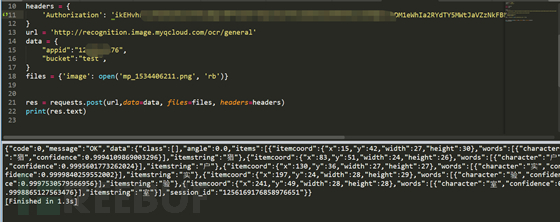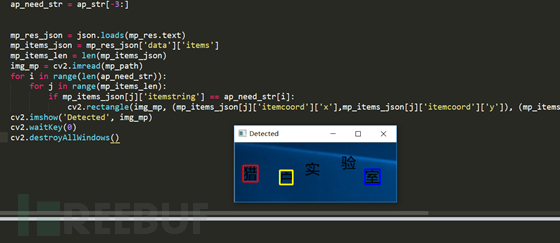## 0×01  验证码的获取

``````<?php
function createImage(\$word    ,\$imagePath,\$type    , \$imageName){
\$fontPath = 'msyh.ttc'    ;//字体
\$fontSize = 20     * 0.75;
foreach (\$word     as \$v ) {
\$fontarea  =     imagettfbbox(\$fontSize,     0 , \$fontPath, \$v        );
\$textWidth =     \$fontarea -     \$fontarea ;
\$textHeight =     \$fontarea -     \$fontarea ;
\$tmp[    'text'] = \$v;
\$tmp[    'size'] = \$fontSize;
\$tmp[    'width'] = \$textWidth;
\$tmp[    'height'] = \$textHeight;
\$textArr[] =     \$tmp;
}
list( \$imageWidth    , \$imageHeight,  \$imageType) =         getimagesize( \$imagePath);
for( \$i    =0;\$i <        count(\$textArr );\$i            ++){
list(    \$x, \$y) =  randPosition        (\$textArr,  \$imageWidth,             \$imageHeight, \$textArr[\$i                ][ 'width'], \$textArr[                    \$i]['height'],                    \$i,\$type);
\$textArr[    \$i]['x'] =     \$x;
\$textArr[    \$i]['y'] =     \$y;
}
unset( \$v    );
//创建图片的实例
\$image =  imagecreatefromstring    (file_get_contents( \$imagePath));
//字体颜色
\$color =  imagecolorallocate    (\$image,  0,         0,  0);
//绘画文字
foreach( \$textArr     as \$v){
imagefttext (    \$image, \$v ['size'        ], 0 , \$v[            'x' ], \$v['y'                ], \$color,  \$fontPath                ,\$v ['text']);
}
if (imagepng    ( \$image,\$imageName )){
echo \$imageName    ." \n";
}
}
functionrandPosition (\$textArr    ,  \$imgW, \$imgH ,         \$fontW,  \$fontH,\$i            ,\$type){
switch  (\$type    ) {
case 0    :// 生成mp
\$x =     rand (\$i* 60        , (\$i +1)*            60-\$fontW -            3);
\$y =     rand (40, 80        );
break;
case1    : //生成ap
\$x = (    \$i )*25+ 5        ;
\$y =     25;
default:
break;
}
\$return =  array    ( \$x,  \$y);
return\$return ;
}
\$ap_imagePath  =  'ap_bg.png' ;
\$mp_imagePath  =  'mp_bg.png' ;
\$ap_imageName  =  "ap_" .    time (). ".png" ;
\$mp_imageName  =  "mp_" .    time (). ".png" ;
\$ap_word  =  array (    ' 请 ' ,    ' 依 ' ,    ' 次 ' ,    ' 点 ' ,    ' 击 ' ,    ' 图 ' ,    ' 中 ' ,    ' 的 ' ,    ' 猎 ' ,     ' 户 ' ,    ' 室 ' )                                                                                                                                                                                                                                                                                                                                                                                                                                                ;
\$mp_word  =  array (    ' 猎 ' ,     ' 户 ' ,     ' 实 ' ,    ' 验 ' ,    ' 室 ' );
createImage ( \$ap_word ,    \$ap_imagePath , 1 ,    \$ap_imageName );
createImage ( \$mp_word ,    \$mp_imagePath , 0 ,    \$mp_imageName );
?>``````

ap_XXXXX.png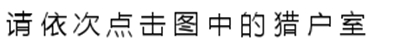mp_XXXXX.png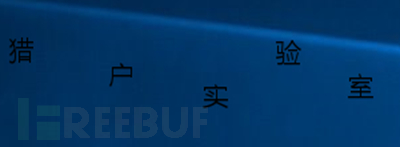ap_XXXXX.png是说明需要点击的文字，mp_XXXXX.png是需要点击的图片。

## 0×02  验证码识别

### 1. opencv的图像模板匹配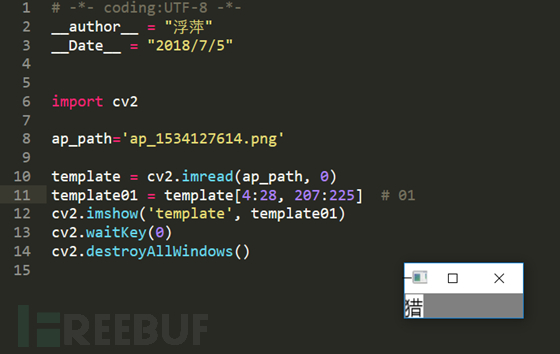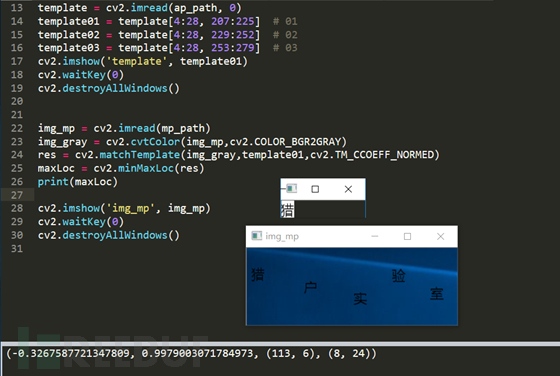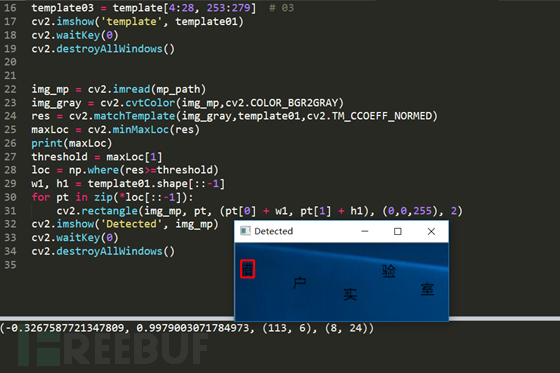``   switch(\$type) {    case0: //mp文字随机大小    \$fontSize=rand (20,30) *0.75 ;    break;    case1 ://ap文字固定    \$fontSize=20 *0.75;    break;       }``

ap生成的结果还是和之前一样， mp 的图片如下：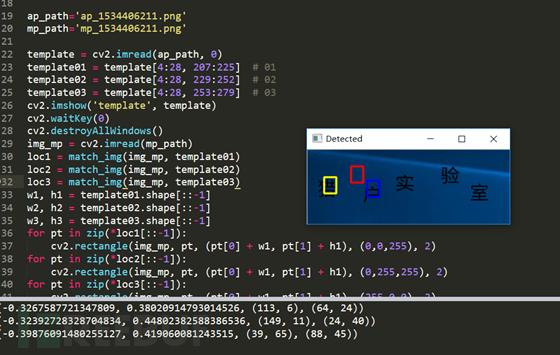### 2.OCR识别